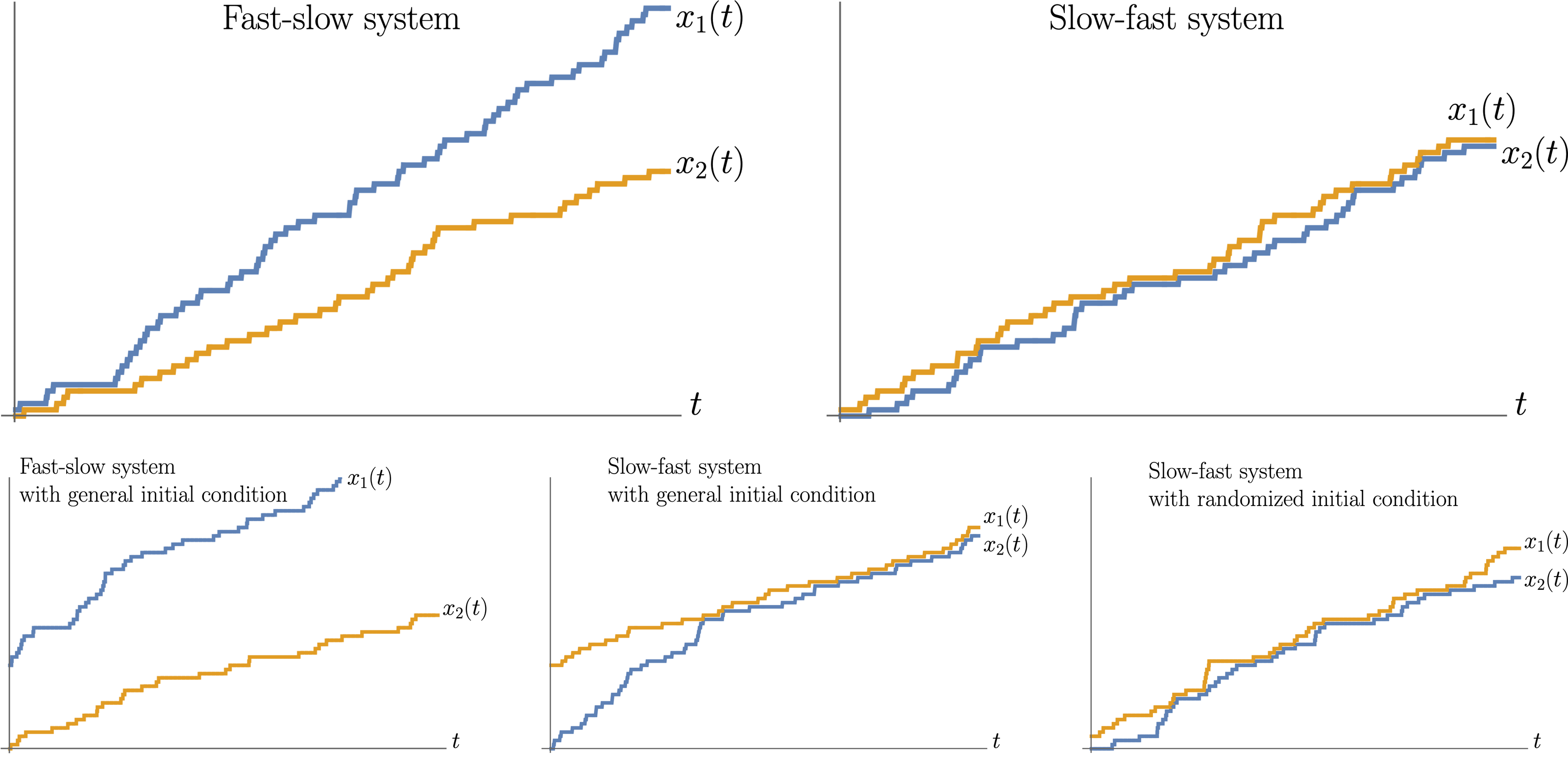# Rewriting History in Integrable Stochastic Particle Systems

### 2022/12/04

Leonid Petrov, Axel Saenz
arXiv:2212.01643 [math.PR]

PDF

Source

Many integrable stochastic particle systems in one space dimension (such as TASEP — Totally Asymmetric Simple Exclusion Process — and its $q$-deformation, the $q$-TASEP) remain integrable if we equip each particle with its own speed parameter. In this work, we present intertwining relations between Markov transition operators of particle systems which differ by a permutation of the speed parameters. These relations generalize our previous works , , but here we employ a novel approach based on the Yang-Baxter equation for the higher spin stochastic six vertex model. Our intertwiners are Markov transition operators, which leads to interesting probabilistic consequences.

First, we obtain a new Lax-type differential equation for the Markov transition semigroups of homogeneous, continuous-time versions of our particle systems. Our Lax equation encodes the time evolution of multipoint observables of the $q$-TASEP and TASEP in a unified way, which may be of interest for the asymptotic analysis of multipoint observables of these systems.

Second, we show that our intertwining relations lead to couplings between probability measures on trajectories of particle systems which differ by a permutation of the speed parameters. The conditional distribution for such a coupling is realized as a “rewriting history” random walk which randomly resamples the trajectory of a particle in a chamber determined by the trajectories of the neighboring particles. As a byproduct, we construct a new coupling for standard Poisson processes on the positive real half-line with different rates.

##### A poem on the topic

by OpenAI

In stochastic particle systems, there’s a way
To rewrite history with each passing day.
A single particle, its fate made clear,
Can undo what’s been done and make it reappear.

The laws of probability and chaos at play
Can be bent to our will, if we but obey.
The deterministic systems in our control,
Will yield to a new order, as it starts to unfold.

The particles and their interactions will dictate,
The outcome of our systems, no matter their state.
With the tools of integrability, we can rewrite,
The future of our systems with a single bite.Two-car systems with cars of different speeds. In every picture, the blue and the yellow trajectories are of the faster and the slower cars, respectively.
Top: cars start at neighboring locations, and the trajectory of the second car, $x_2(t)$, is the same in distribution.
Bottom: cars start at locations away from each other, and the distributions of the trajectories of the second car are different in the left and the central pictures. However, when we randomize the initial condition, the distribution of the second car in the slow-fast system with the randomized initial condition is the same as in the fast-slow system on the left.